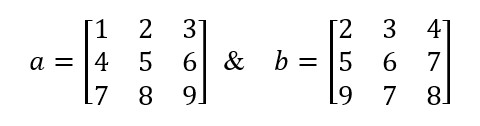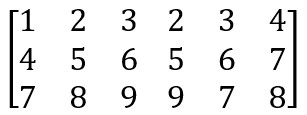# Problem 55480. Array Concatenation (1)

Given two matrices, a and b, concatenate the two matrices horizontally, i.e., the number of columns of the result should be equal to the sum of the number of columns of matrix a and matrix b. Assume both matrices a and b have the the same number of rows and the result will also have the same number of rows.
For example, ifThe result should be### Solution Stats

63.24% Correct | 36.76% Incorrect
Last Solution submitted on Dec 01, 2023

### Community Treasure Hunt

Find the treasures in MATLAB Central and discover how the community can help you!

Start Hunting!